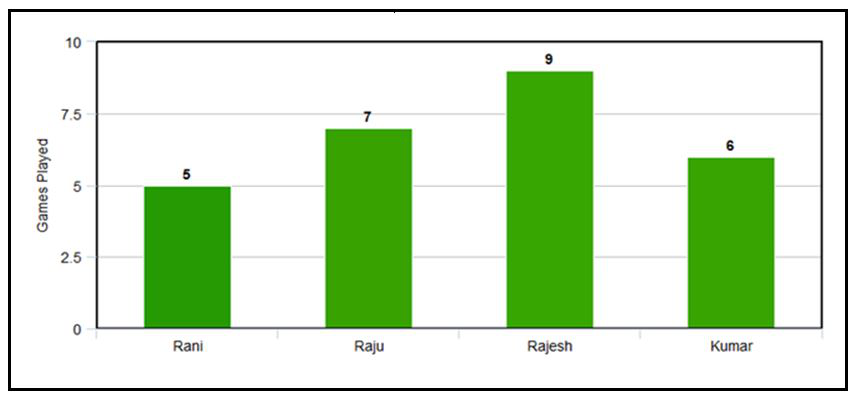# Mean, Median, Mode, and Range – Statistics | Class 9 Maths

• Difficulty Level : Medium
• Last Updated : 28 Dec, 2020

A central tendency is a single value or average that represents the whole set of data. It is referred to as the central location of data distribution. The value that is represented as a central tendency is within the range of the data. There are 3 measures of central tendency. They are:

1. Mean (x̅ or μ)
2. Median(M)
3. Mode(Z)

## Mean

Mean is the sum of all the values in the data set divided by the number of values in the data set. It is also called the Arithmetic Average. If x1, x2, x3,……, xn are the values of a data set then the mean is denoted as x̅ (x bar) and the formula is calculated as:

### x̅ =  (x1 + x2 + x3 + …… + xn) / n

Example:

The mean of the data 10, 30, 40, 20, 50 is

Mean = (sum of all data values) / (number of values)

Mean = (10 + 30 + 40 + 20+ 50) / 5=30

## Median

A Median is a middle value for a sorted data. The sorting of the data can be done either in ascending order or in descending order. A median divides the data into two halves. The formula for median:

If the number of values (n value) in the data set is odd then the formula to calculate median is:

### Median = ((n + 1)/2)th term

If the number of values (n value) in the data set is even then the formula to calculate median is:

### Median  = [(n/2)th term + {(n/2) + 1}th term] / 2

Example 1:

The median of the data 30, 40, 10, 20, 50 is:

Step 1: Order the given data in ascending order as:

10, 20, 30, 40, 50

Step 2: Check n (number of terms of data set) is even or odd and find the median of the data with respective ‘n’ value.

Step 3: Here, n = 5 (odd) then Median = [(n + 1)/2]th term 10, 20, 30, 40, 50

The median of the data is [(5 + 1)/2]th term is 30.

Example 2:

25, 12, 5, 24, 15, 22, 23, 25

Step 1: Order the given data in ascending order as:

5, 12, 15, 22, 23, 24, 25, 25

Step 2: Check n (number of terms of data set) is even or odd and find the median of the data with respective ‘n’ value.

Step 3: Here, n = 8 (even) then,

Median = [(n/2)th term + {(n/2) + 1)th term] / 2

Median = [(8/2)th term + {(8/2) + 1}th term] / 2 = (22+23) / 2 = 22.5

## Mode

A mode is the most frequent value or item of the data set. A data set can generally have one or more than one mode value. If the data set has one mode then it is called “Uni-modal”. Similarly, If the data set contains 2 modes then it is called “Bimodal” and if the data set contains 3 modes then it is known as “Trimodal”. If the data set consists of more than one mode then it is known as “multi-modal”(can be bimodal or trimodal). There is no mode for a data set if every number appears only once.

Example 1:

If the data set is {1, 2, 2, 3, 3, 4, 5} then it has 2 modes i.e, 2 and 3 (bi-modal). Since, both the values 2 and 3 are repeating twice in the data set.

Example 2:

If the data set is {15, 42, 65, 65, 95} then the mode is 65 (uni-modal). Since 65 is the only repeating value in the data set.

## Range

It is the difference between the highest value and the lowest value. It is a way to understand how the numbers are spread in a data set. Formula to find Range is:

### Range = Highest value – Lowest Value

Example:

If the data set is {12, 19, 6, 2, 15, 4} then the lowest value is 2 and the highest value is 19.

So the range is 19 − 2 = 17.

## Reading Bar Charts: Putting it Together with Central TendencyQuestion 1. Finding Mean for the above bar chart.

Mean = (sum of all data values) / (number of values)

Mean = (5 + 7 + 9 + 6) / 4  =  27 / 2 = 6.75

Question 2. Finding the Median for the above bar chart:

Order the given data in ascending order as: 5, 6, 7, 9

Here, n = 4 (number of students which is even)

Median  =  [(n/2)th term + {(n/2) + 1}th term] / 2

Median  = (6 + 7) / 2  =  6.5

Question 3. Finding Mode for the above bar chart:

Mode = most frequent value = 9 (highest value)

Question 4. Finding the range for the above bar chart:

Range = highest value – lowest value

Range = 9 – 5 = 4

My Personal Notes arrow_drop_up